# Daily temperature

The average of daily temperature measurements in one week every day at the same hour was -2.8 °C. We measured all temperatures on different days are different. The highest daily maximum temperature was 2.4 °C, the lowest -6 °C. Determine the options that We can measure temperature in the remaining five days.

a =  -3.4 °C
b =  -3.3 °C
c =  -3.2 °C
d =  -3.1 °C
f =  -3 °C

### Step-by-step explanation:

$b=a+0.1=\left(-3.4\right)+0.1=-3.3\text{°C}$
$c=b+0.1=\left(-3.3\right)+0.1=-3.2\text{°C}$
$d=c+0.1=\left(-3.2\right)+0.1=-3.1\text{°C}$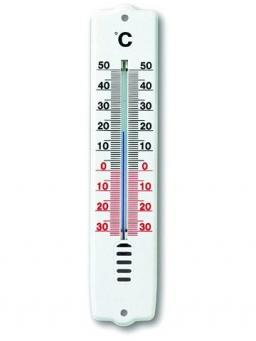Did you find an error or inaccuracy? Feel free to write us. Thank you!Tips to related online calculators
Looking for help with calculating arithmetic mean?
Looking for a statistical calculator?
Do you want to convert time units like minutes to seconds?

#### You need to know the following knowledge to solve this word math problem:

We encourage you to watch this tutorial video on this math problem:

## Related math problems and questions:

• The temperature 13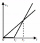The temperature in Toronto at noon during a winter day measured 4°C. The temperature started dropping 2° every hour. Which inequality can be used to find the number of hours, x, after which the temperature will measure below -3°C?
• Daily average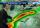Calculate the average temperature during the day, when 15 hours were 20 °C and 9 hours was 13 °C.
• The lowestThe lowest temperature on Thursday was 20°C. The lowest temperature on Saturday was 12°C. What was the difference between the lowest temperatures?
• Degrees FahrenheitC= (5)/(9)(F−32) The equation above shows how temperature F, measured in degrees Fahrenheit, relates to a temperature C, measured in degrees Celsius. Based on the equation, which of the following must be true? I. A temperature increase of 1 degree Fahrenh
• Temperature difference 2The temperature in London on new year’s day is -2 degree Celsius. The temperature in Moscow on the same day is -14 degree Celsius, what is the temperature difference between the two cities?
• The temp rangeWhat is the range in temperatures if the high temperatures is 67 degrees Celsius and the low temperature is -25 degrees Celsius?
• Difference of temperaturesThe temperature on Saturday was –2°C. The temperature on Sunday was 8°C. Write down the difference between these two temperatures.
• Equal temperature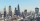The temperatures of the two cities were measured at the same time. The temperature in city A was 60 degrees And rose at a constant rate of 2 degrees per hour. The temperature in city B was 40° and rose at a constant rate of 10° per hour Enter the time in
• Change in temperatureStarting temperature is 21°C, the highest temperature is 32°C. What is the change in temperature?
• Water temperaturesTo 25 liters of water at 50 °C we add 15 liters of water at a different temperature. How many °C should the water be colder than 50 °C so that 40 liters of the water obtained has a temperature 42.5 °C?
• Temperature up and dowm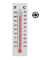At 6 AM, the temperature is 19.4°C. Between 6 AM and noon, the temperature rises 3.8°C. Between noon and 6 PM, the temperature falls 2.5°C. What is the temperature at 6 PM?
• The temperature 14The temperature is 12°C lower than 4 hours ago. a. What is the average temperature change per hour? b. Write an integer equation for the situation.
• WeatherWeather. At 5:00 am, the temperature was 28°C, and at noontime, it rose by 9°C. By 4:00 pm, it dropped 3°C, and by nighttime, it was 4°C less. What is the room temperature this time?
• Temperature differenceLibya's highest temperature was recorded at 58 degrees Celsius, and the lowest was recorded at -88 degrees Celsius. What is the temperature difference?
• The poolThe pool has a volume of 40 m3, and the water temperature is 20 °C. How much water at 100 °C should we pour into the pool to increase the water temperature by 5 °C?
• The temperature 12The temperature of a liquid was 25°C before it was warmed at a rate of 10°C per minute for 5 minutes. It was then cooled at 3.5°C per minute for 6 minutes. What was the temperature of the liquid after cooling?
• Temperature changeThe mean temperature change is -3.2°F per day for five days. What is the total change over the five day period?